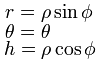# Spherical coordinate system – 구 좌표계In mathematics, the spherical coordinate system is a coordinate system for representing geometric figures in three dimensions using three coordinates: the radial distance of a point from a fixed origin, the zenith angle from the positive z-axis, and the azimuth angle from the positive x-axis.

Definition
The three coordinates (ρ, θ, φ) are defined as:

* ρ ≥ 0 is the distance from the origin to a given point P.
* 0 ≤ θ ≤ 360° is the angle between the positive x-axis and the line from the origin to the P projected onto the xy-plane.
* 0 ≤ φ ≤ 180° is the angle between the positive z-axis and the line formed between the origin and P.

θ is referred to as the azimuth, while φ is referred to as the zenith, colatitude or polar angle.
θ and φ and lose significance when ρ = 0 and θ loses significance when sin(φ) = 0 (at φ = 0 and φ = 180°).

To plot a point from its spherical coordinates, go ρ units from the origin along the positive z-axis, rotate φ about the y-axis in the direction of the positive x-axis and rotate θ about the z-axis in the direction of the positive y-axis.

Coordinate system conversions
As the spherical coordinate system is only one of many three-dimensional coordinate systems, there exist equations for converting coordinates between the spherical coordinate system and others.

Cartesian coordinate system
The three spherical coordinates are obtained from Cartesian coordinates by:Note that the arctangent must be defined suitably so as to take account of the correct quadrant of y / x. The atan2 or equivalent function accomplishes this for computational purposes.Cylindrical coordinate system

The cylindrical coordinate system is a three-dimensional extrusion of the polar coordinate system, with an h coordinate to describe a point’s height above or below the xy-plane. The full coordinate tuple is (r, θ, h).

Cylindrical coordinates may be converted into spherical coordinates by:Spherical coordinates may be converted into cylindrical coordinates by:출처: 위키백과